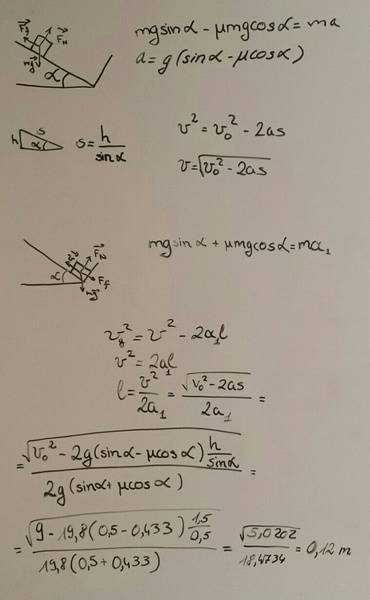# Moving body on an inclined plane

## Homework Statement

A body is moving on an inclined plane which has a slope of 30o. When the body is h=1,5m above the surface it has a velocity of vo=3 m/s. At the bottom of the plane it bounces elastically and starts moving upwards. Find the height it reaches, when the coefficient of frictions is μ=0.5, g=9.8 m/s2

## Homework Equations

Newton's laws of motion

## The Attempt at a SolutionThe correct answer is 0.35 m. Please say where is my mistake.

## Answers and Replies

If I were you, I would try using energy instead of forces. I think it will make your life much easier.

Good day. Why don't you try using: (body's gravitational potential energy) +(it's kinetic energy) = ( kinetic energy at the bottom of the incline) to find the energy at the bottom on the ramp. Then use that energy to find the height the box reaches by subtracting work done by friction and setting it equal to gravitational P.E then solve for h.

TSny
Homework Helper
Gold Member
For the block going down you wrote v2 = v02 - 2as. Why the minus sign?

For the block going up you have l = v2/(2a1). Did you forget to square the velocity when you substituted for v?

2*9.8 = 19.6 rather than 19.8

You are asked for the final height rather than the final distance along the incline.

Everything turned out fine. Thanks for the help.

Everything turned out fine. Thanks for the help.
Would you like kind enough to say what was wrong?

Has anyone been able to get the answer that the OP says was correct? I have done this problem with both paradigms to try amd help them however I can not see how this answer of .35m is reached. Both ways give me that the mass hits at 3.597 m/s.

TSny
Homework Helper
Gold Member
Has anyone been able to get the answer that the OP says was correct? I have done this problem with both paradigms to try amd help them however I can not see how this answer of .35m is reached. Both ways give me that the mass hits at 3.597 m/s.
I get 0.35 m for the answer using the OP's method and also using energy concepts.

I agree with you that the block reaches the bottom with a speed of 3.597 m/s.

I get 0.35 m for the answer using the OP's method and also using energy concepts.

I agree with you that the block reaches the bottom with a speed of 3.597 m/s.

After that don't you just square that velocity then divide by two times 9.8?

TSny
Homework Helper
Gold Member
After that don't you just square that velocity then divide by two times 9.8?
Ah. I think it's an interpretation problem. The problem statement says that at the bottom it "bounces elastically and starts moving upward". Apparently this was supposed to mean that the block bounces back up along the incline rather than vertically upward. (Look at the "wall" at the bottom in the OP's diagram.)

•FallenLeibniz
Ah. I think it's an interpretation problem. The problem statement says that at the bottom it "bounces elastically and starts moving upward". Apparently this was supposed to mean that the block bounces back up along the incline rather than vertically upward. (Look at the "wall" at the bottom in the OP's diagram.)
Riiight. That makes more sense than the block jumping up in the air. Oops.